Homework Help Question & Answers

# KITES DE INSTALA (2) Given the following grammar, E ::= E + F E ::= F:...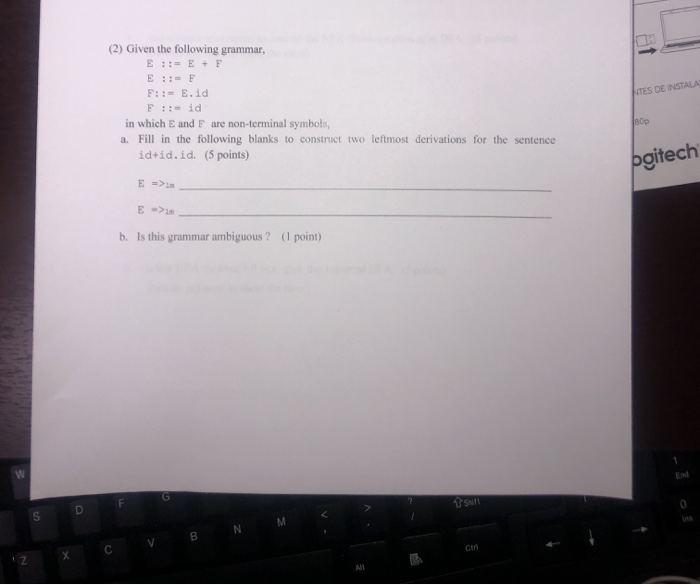KITES DE INSTALA (2) Given the following grammar, E ::= E + F E ::= F: := E.id F ::= id in which E and F are non-terminal symbols, a. Fill in the following blanks to construct two leftmost derivations for the sentence id+id.id. (5 points) 80p pgitech E -> E -> b. Is this grammar ambiguous ? (1 point) N v B c iz X

#### Homework Answers

Answer #1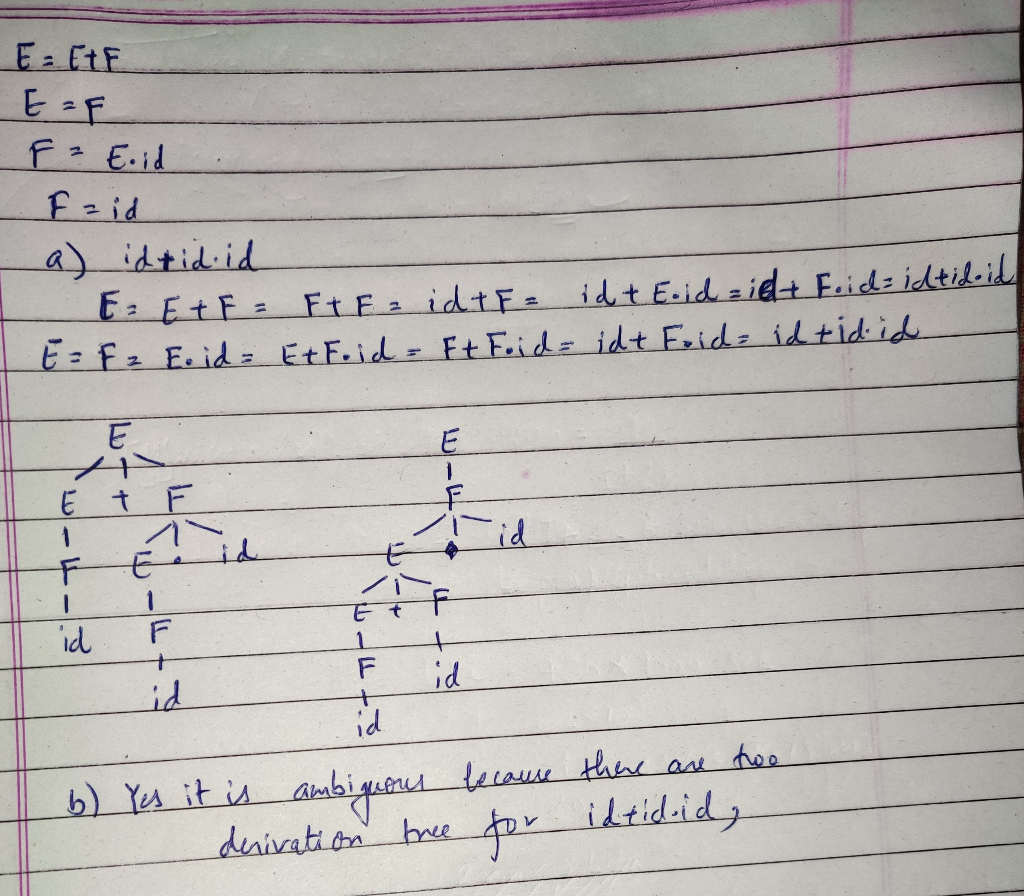Know the answer?
Your Answer:

#### Post as a guest

Your Name:

What's your source?

#### Earn Coin

Coins can be redeemed for fabulous gifts.

Not the answer you're looking for? Ask your own homework help question. Our experts will answer your question WITHIN MINUTES for Free.
Similar Homework Help Questions
• ### Consider the following grammar (G1) for simple assignment statements. (The symbols in double quotation marks are...

Consider the following grammar (G1) for simple assignment statements. (The symbols in double quotation marks are terminal symbols.) assign → id “ = ” expr id → “A” | “B” | “C” expr → expr “ + ” expr | expr “ ∗ ” expr | “(” expr “)” | id a) Give a (leftmost) derivation for string A = B ∗ A + C. b) Give the parse tree for string A = B ∗ A + C. c)...

• ### Given the following ambiguous context free grammar (3x20) 1. (a) Explain why the grammar is ambiguous...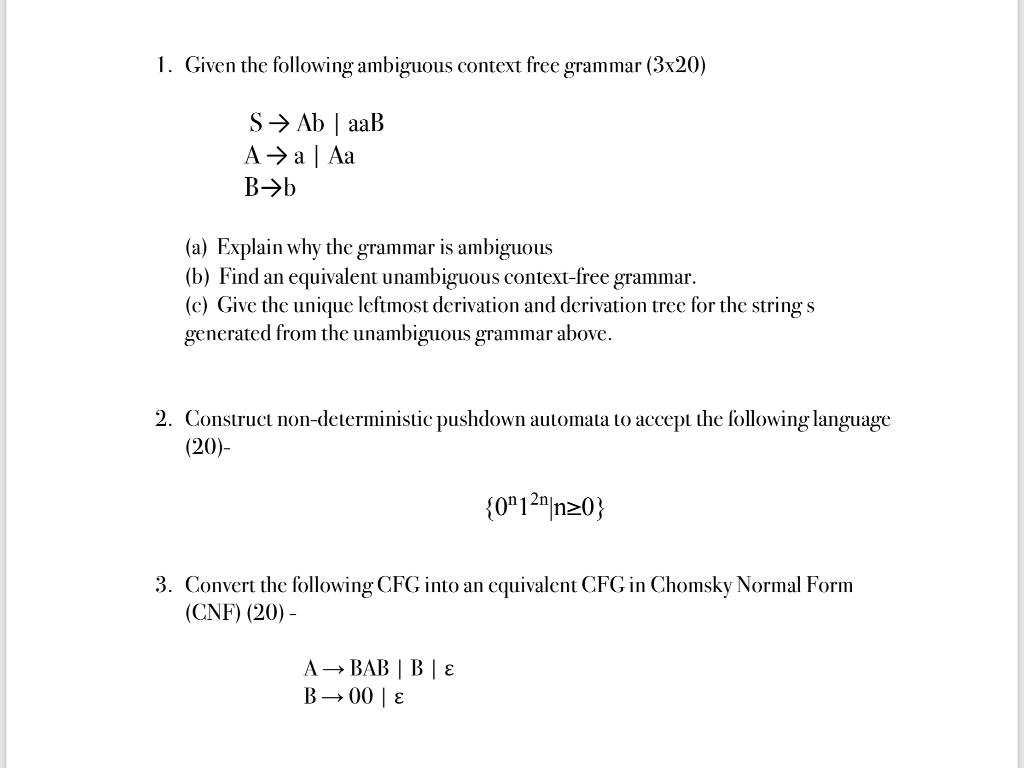Given the following ambiguous context free grammar (3x20) 1. (a) Explain why the grammar is ambiguous (b) Find an equivalent unambiguous context-free grammar. (c) Give the unique leftmost derivation and derivation tree for the string s generated from the unambiguous grammar above. 2. Construct non-deterministic pushdown automata to accept the following language (20) 3. Convert the following CFG into an cquivalent CFG in Chomsky Normal Form (CNF) (20)-

• ### 1.)    Consider the following grammar in which S, A, and B are nonterminal symbols and S...

1.)    Consider the following grammar in which S, A, and B are nonterminal symbols and S is the start symbol. S → 1A | 0B A → A0 | 1B B → 10A| 1 Show that the grammar is ambiguous by showing two parse trees for the sentence 1110110 using leftmost derivation.

• ### Consider the following grammar (S, A, B, and C are nonterminal symbols; S is the start...

Consider the following grammar (S, A, B, and C are nonterminal symbols; S is the start symbol; 0 and 1 are terminal symbols): S → AA A → BCB B → B0 | B1 | 0 | 1 C → 00 | 11 Which of the following sentences are in the language generated by the grammar? Show derivations for the sentences that can be generated. If a sentence cannot be generated by the grammar, explain why. a) 10010001 b) 01101101...

• ### (a0Give the derivations and parse trees for the following strings using the grammar given below: •...

(a0Give the derivations and parse trees for the following strings using the grammar given below: • abba • babab (b)   Give the derivations and parse trees for the following strings using the grammar given below: • a cat napped • a cat barked P={ <sentence> → <article><noun><verb> <article> → "a" <article> → "the" <noun → "dog" <noun> → "cat" <verb> → "barked" <verb> → "napped" }

• ### Question Set 2 1. Given the following grammar dactor>-> ( <expr> ) a) What is the...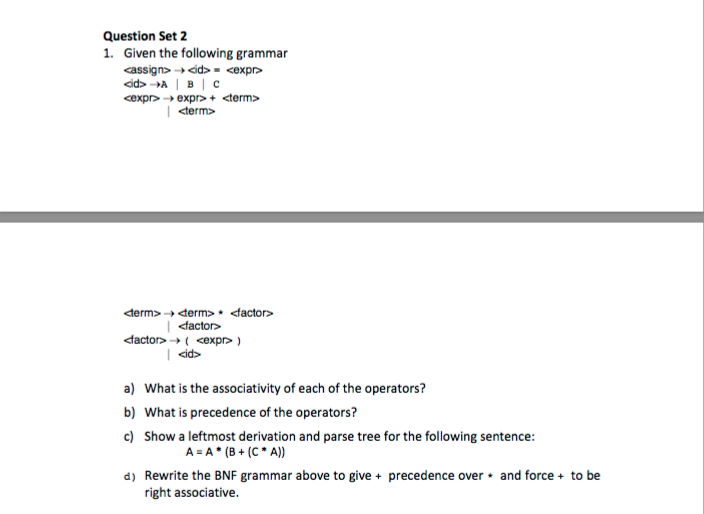Question Set 2 1. Given the following grammar dactor>-> ( <expr> ) a) What is the associativity of each of the operators? What is precedence of the operators? Show a leftmost derivation and parse tree for the following sentence: b) c) A-A(B(C A)) d) Rewrite the BNF grammar above to give precedence over and force to be right associative.

• ### Question Set 2 1. Given the following grammar dactor>-> ( <expr> ) a) What is the...Question Set 2 1. Given the following grammar dactor>-> ( <expr> ) a) What is the associativity of each of the operators? What is precedence of the operators? Show a leftmost derivation and parse tree for the following sentence: b) c) A-A(B(C A)) d) Rewrite the BNF grammar above to give precedence over and force to be right associative.

• ### Consider following note that are terminals. E rightarrow e + t | t t rightarrow ID...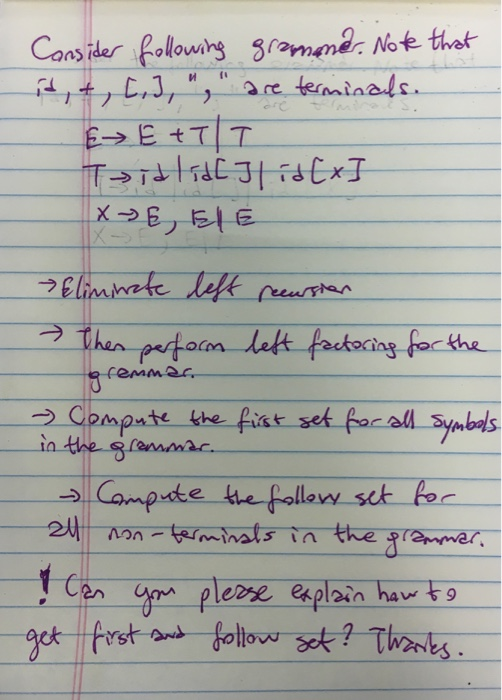Consider following note that are terminals. E rightarrow e + t | t t rightarrow ID | j x rightarrow e, E | E Eliminate left Then perform left factoring for the grammar Compute the first set for all symbols in the grammar Compute the follow set for non-terminal in the grammar. Can you please explain how to get first follow set? Thanks.

• ### The questions in this section are based on the grammar given as the following: prog ->...

The questions in this section are based on the grammar given as the following: prog -> assign | expr assign -> id = expr expr -> expr + term | expr - term | term term -> factor | factor * term factor -> ( expr ) | id | num id -> A | B | C num -> 0 | 1 | 2 | 3 (2a) What is the associativity of the * operator? (5 points) (2b) What...

• ### Q6) Consider the following grammar for arithmetic expressions. F ? (E) l i Using top-down parsing,...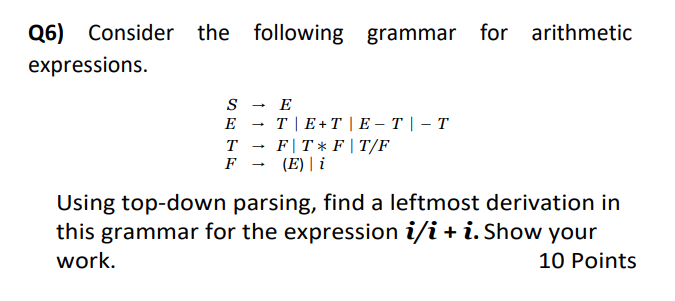Q6) Consider the following grammar for arithmetic expressions. F ? (E) l i Using top-down parsing, find a leftmost derivation in this grammar for the expression i/i + . Show your work. 10 Points